# C++ 虚函数表解析

+关注继续查看

（虽然转的，但是文章确实不错，所以还是脸皮厚点发到首页......，管理员，你看着办吧！！！）

## 前言

C++中的虚函数的作用主要是实现了多态的机制关于多态，简而言之就是用父类型别的指针指向其子类的实例，然后通过父类的指针调用实际子类的成员函数。这种技术可以让父类的指针有“多种形态”，这是一种泛型技术。所谓泛型技术，说白了就是试图使用不变的代码来实现可变的算法。比如：模板技术，RTTI技术，虚函数技术，要么是试图做到在编译时决议，要么试图做到运行时决议。

### 虚函数表

C++ 了解的人都应该知道虚函数（Virtual Function）是通过一张虚函数表（Virtual Table）来实现的。简称为V-Table。在这个表中，主是要一个类的虚函数的地址表，这张表解决了继承、覆盖的问题，保证其容真实反应实际的函数。这样，在有虚函数的类的实例中这个表被分配在了这个实例的内存中，所以，当我们用父类的指针来操作一个子类的时候，这张虚函数表就显得由为重要了，它就像一个地图一样，指明了实际所应该调用的函数。

class Base {
public:
virtual void f() { cout << "Base::f" << endl; }
virtual void g() { cout << "Base::g" << endl; }
virtual void h() { cout << "Base::h" << endl; }

};

 typedef void(*Fun)(void);

Base b;

Fun pFun = NULL;

cout << "虚函数表地址：" << (int*)(&b) << endl;
cout << "虚函数表 — 第一个函数地址：" << (int*)*(int*)(&b) << endl;

// Invoke the first virtual function
pFun = (Fun)*((int*)*(int*)(&b));
pFun();

Base::f

   (Fun)*((int*)*(int*)(&b)+0);  // Base::f()
(Fun)*((int*)*(int*)(&b)+1);  // Base::g()
(Fun)*((int*)*(int*)(&b)+2);  // Base::h()### 一般继承（无虚函数覆盖）// CppTest.cpp : 定义控制台应用程序的入口点。
//

#include "stdafx.h"
#include <iostream>
using namespace std;

class Base{
public:
virtual void f(){cout<<"Base::f\n";}
virtual void g(){cout<<"Base::g\n";}
virtual void h(){cout<<"Base::h\n";}
};

class Derive:public Base{

public:
virtual void f1(){cout<<"Derive:f1\n";}
virtual void g1(){cout<<"Derive:g1\n";}
virtual void h1(){cout<<"Derive:h1\n";}
};

int _tmain(int argc, _TCHAR* argv[])
{
typedef void(*FUN)(void);

Base b;
FUN pFun=NULL;

cout<<"虚函数表的地址为："<<(int*)(&b)<<endl;
cout << "虚函数表 — 第一个函数地址：" << (int*)*(int*)(&b) << endl;
for (int i=0;i<3;++i)
{
pFun = (FUN)*((int*)*(int*)(&b)+i);
pFun();
}

cout<<"-----------------------------------------"<<endl;

Derive d;
pFun=NULL;

cout<<"虚函数表的地址为："<<(int*)(&d)<<endl;
cout<<"虚函数表 — 第一个函数地址："<<(int*)*(int*)(&d)<<endl;

for (int i=0;i<6;++i)
{
pFun=(FUN)*((int*)*(int*)(&d)+i);
pFun();
}

return 0;
}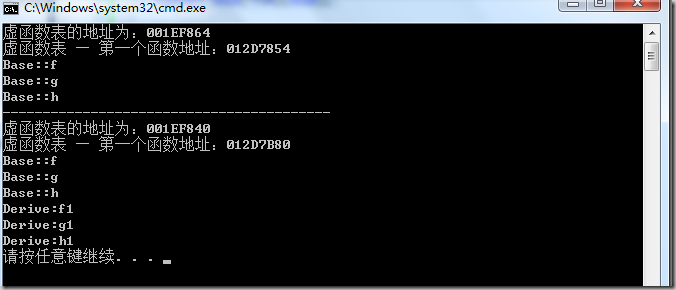1）虚函数按照其声明顺序放于表中。

2）父类的虚函数在子类的虚函数前面。

## 一般继承（有虚函数覆盖）#include <iostream>
using namespace std;

class Base{
public:
virtual void f(){cout<<"Base::f\n";}
virtual void g(){cout<<"Base::g\n";}
virtual void h(){cout<<"Base::h\n";}
};

class Derive:public Base{

public:
virtual void f(){cout<<"Derive:f\n";}
virtual void g1(){cout<<"Derive:g1\n";}
virtual void h1(){cout<<"Derive:h1\n";}
};

int _tmain(int argc, _TCHAR* argv[])
{
typedef void(*FUN)(void);

Base b;
FUN pFun=NULL;

cout<<"虚函数表的地址为："<<(int*)(&b)<<endl;
cout << "虚函数表 — 第一个函数地址：" << (int*)*(int*)(&b) << endl;
for (int i=0;i<3;++i)
{
pFun = (FUN)*((int*)*(int*)(&b)+i);
pFun();
}

cout<<"-----------------------------------------"<<endl;

Derive d;
pFun=NULL;

cout<<"虚函数表的地址为："<<(int*)(&d)<<endl;
cout<<"虚函数表 — 第一个函数地址："<<(int*)*(int*)(&d)<<endl;

for (int i=0;i<5;++i)
{
pFun=(FUN)*((int*)*(int*)(&d)+i);
pFun();
}

return 0;
}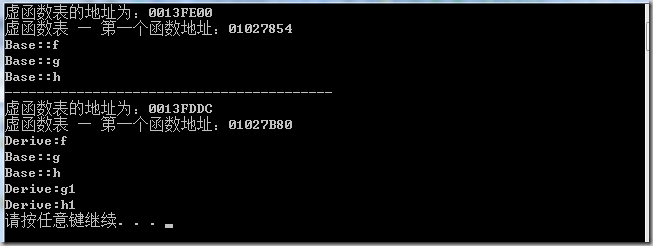1）覆盖的f()函数被放到了虚表中原来父类虚函数的位置。

2）没有被覆盖的函数依旧。

Base *b = new Derive();

b->f();

### 多重继承（无虚函数覆盖）#include <iostream>
using namespace std;

class Base1{
public:
virtual void f(){cout<<"Base1::f\n";}
virtual void g(){cout<<"Base1::g\n";}
virtual void h(){cout<<"Base1::h\n";}
};

class Base2{
public:
virtual void f(){cout<<"Base2::f\n";}
virtual void g(){cout<<"Base2::g\n";}
virtual void h(){cout<<"Base2::h\n";}
};

class Base3{
public:
virtual void f(){cout<<"Base3::f\n";}
virtual void g(){cout<<"Base3::g\n";}
virtual void h(){cout<<"Base3::h\n";}
};

class Derive:public Base1,Base2,Base3{

public:
virtual void f1(){cout<<"Derive:f1\n";}
virtual void g1(){cout<<"Derive:g1\n";}
virtual void h1(){cout<<"Derive:h1\n";}
};

int _tmain(int argc, _TCHAR* argv[])
{
typedef void(*FUN)(void);

Derive d;
FUN pFun=NULL;
cout<<"虚函数表的地址为："<<(int*)(&d)<<endl;
cout << "虚函数表 — 第一个函数地址：" << (int*)*(int*)(&d) << endl;

for (int i=0;i<6;++i)
{
pFun = (FUN)*((int*)*(int*)(&d)+i);
pFun();
}

cout<<"----------------------------"<<endl;

for (int i=0;i<3;++i)
{
pFun = (FUN)*((int*)*((int*)(&d)+1)+i);
pFun();
}

cout<<"----------------------------"<<endl;

for (int i=0;i<3;++i)
{
pFun = (FUN)*((int*)*((int*)(&d)+2)+i);
pFun();
}

cout<<"----------------------------"<<endl;

return 0;
}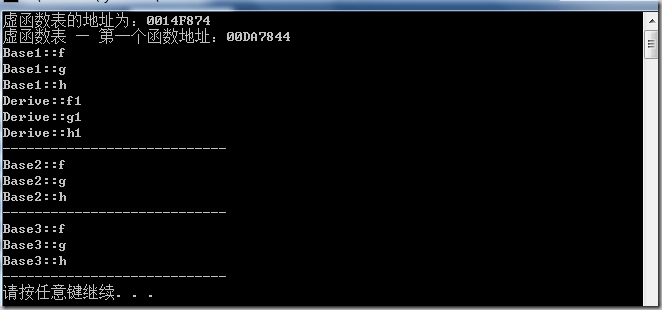### 多重继承（有虚函数覆盖）#include <iostream>
using namespace std;

class Base1{
public:
virtual void f(){cout<<"Base1::f\n";}
virtual void g(){cout<<"Base1::g\n";}
virtual void h(){cout<<"Base1::h\n";}
};

class Base2{
public:
virtual void f(){cout<<"Base2::f\n";}
virtual void g(){cout<<"Base2::g\n";}
virtual void h(){cout<<"Base2::h\n";}
};

class Base3{
public:
virtual void f(){cout<<"Base3::f\n";}
virtual void g(){cout<<"Base3::g\n";}
virtual void h(){cout<<"Base3::h\n";}
};

class Derive:public Base1,Base2,Base3{

public:
virtual void f(){cout<<"Derive::f\n";}
virtual void g1(){cout<<"Derive::g1\n";}
virtual void h1(){cout<<"Derive::h1\n";}
};

int _tmain(int argc, _TCHAR* argv[])
{
typedef void(*FUN)(void);

Derive d;
FUN pFun=NULL;
cout<<"虚函数表的地址为："<<(int*)(&d)<<endl;
cout << "虚函数表 — 第一个函数地址：" << (int*)*(int*)(&d) << endl;

for (int i=0;i<5;++i)
{
pFun = (FUN)*((int*)*(int*)(&d)+i);
pFun();
}

cout<<"----------------------------"<<endl;

for (int i=0;i<3;++i)
{
pFun = (FUN)*((int*)*((int*)(&d)+1)+i);
pFun();
}

cout<<"----------------------------"<<endl;

for (int i=0;i<3;++i)
{
pFun = (FUN)*((int*)*((int*)(&d)+2)+i);
pFun();
}

cout<<"----------------------------"<<endl;

return 0;
}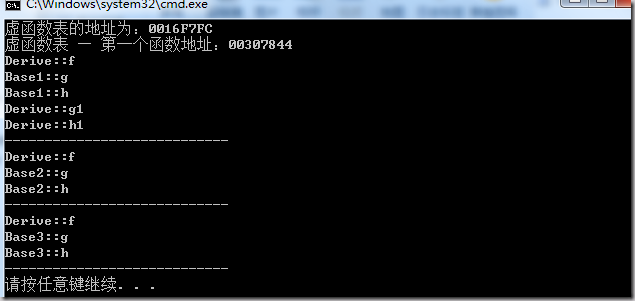Derive d;
Base1 *b1 = &d;
Base2 *b2 = &d;
Base3 *b3 = &d;
b1->f(); //Derive::f()
b2->f(); //Derive::f()
b3->f(); //Derive::f()

b1->g(); //Base1::g()
b2->g(); //Base2::g()
b3->g(); //Base3::g(

### 安全性

 Base1 *b1 = new Derive();
b1->f1();  //编译出错


#include <iostream>
using namespace std;

class Base {
private:
virtual void f() { cout << "Base::f" << endl; }

};

class Derive : public Base{

};

int _tmain(int argc, _TCHAR* argv[])
{
typedef void(*Fun)(void);

Derive d;
Fun  pFun = (Fun)*((int*)*(int*)(&d)+0);
pFun();

return 0;
}

### 结束语

C++这门语言是一门Magic的语言，对于程序员来说，我们似乎永远摸不清楚这门语言背着我们在干了什么。需要熟悉这门语言，我们就必需要了解C++里面的那些东西，需要去了解C++中那些危险的东西。不然，这是一种搬起石头砸自己脚的编程语言。

### 附录一：VC中查看虚函数表691 0C++ 虚函数表解析
981 0903 0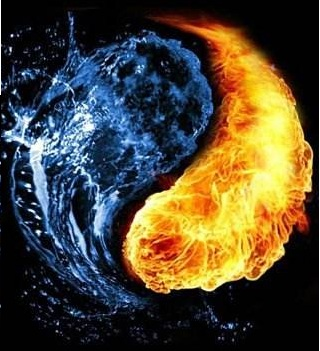C++ 虚函数表解析

1093 0C++之：虚函数表
2802 0（一〇九）单独编译（多个源代码文件和头文件）
3455 0C++ 虚函数表解析

918 0C++虚析构函数解析
当派生类对象从内存中撤销时一般先运行派生类的析构函数，然后再调用基类的析构函数。 如果用new运算符建立的派生类的临时对象，对指向基类的指针指向这个临时对象当用delete运算符撤销对象时，系统执行的是基类的析构函数，而不是派生类的析构函数，不能彻底的“清理现场”。
736 0C++ 虚函数表解析
864 0640 0
406

0